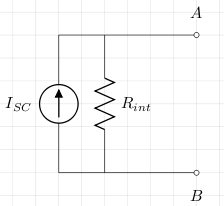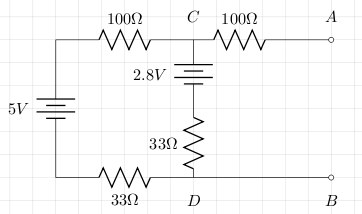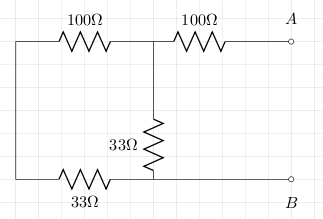# Norton's Theorem

Norton's theorem states that any two terminal voltage source can be represented by a constant current source with internal parallel resistance Rint as in the circuit below:The values for ISC and Rint can either be calculated or measured.

### Calculation

When calculating, first use Kirchhoff's or superposition to compute the open circuit voltage VOC. Next calculate the value of Rint by replacing all voltage sources by their internal resistances. The value of ISCis VOC/Rint.

### Measuring

To measure, first find the short circuit current from A to B and call this ISC. Next measure the open circuit voltage (VOC). The value of Rint is VOC/ISC.

## Example

We'll calculate and measure the values for ISC, VOC and Rint for the circuit below.### Calculation

We can use Kirchhoff's voltage law to give us the current:

```5 - 2.8 = I*(100+33+33)
2.2 = I*166.
I = 2.2/166 = 0.013
```

Now VOC is VCD, so:
VOC = 2.8 + 0.013*33 = 3.23

For Rint we short all voltage sources (assuming no internal resistance) and this gives the following circuit:The calculation of Rint is quite easy:
Rint = 100 + 1/(1/33 + 1/133) = 100 + 26.4 = 126.4

From this we can calculate the short circuit current:
ISC = 3.23 / 126.4 = 0.0255.

### Measurement

The circuit was built and using a multimeter the value of VOC(VCD) was 3.3V. The short circuit current ISC was measured to be 25.6mA. From this we can calculate Rint:
Rint = 3.3/0.0256 = 128.9

### Comparison

The table below shows how close the actual measurements were to the theoretical calculations.

VOC ISC Rint 3.3 0.0256 128.9 3.23 0.0255 126.4

## References

Fischer-Cripps. A.C., The Electronics Companion. Institute of Physics, 2005.